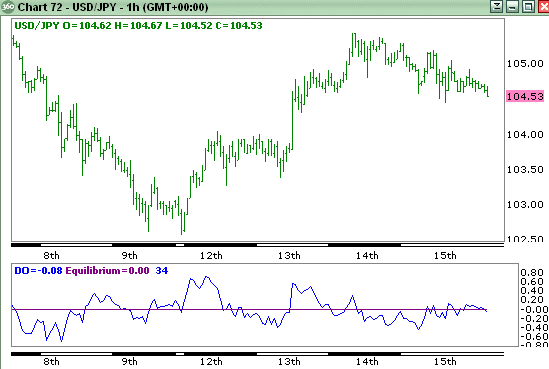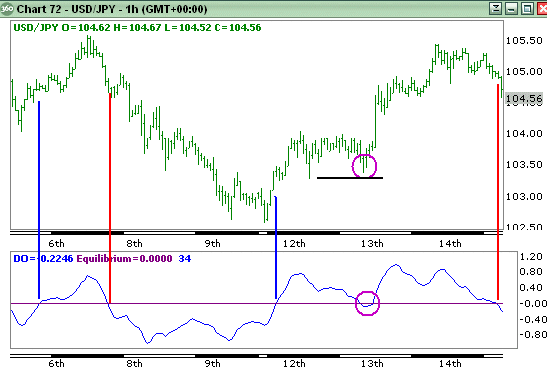### A useful directional oscillator

Have you ever looked for an indicator that could provide you with a broad indication of price direction? Well, here’s a nifty little indicator that could help.

It’s very similar to MACD but tends to suffer fewer whipsaws in flatter corrections. The basic concept behind the MACD indicator is instead of using the crossover signals of two moving averages to base signals, it assigns one (exponential) moving average to represent price and a second, longer (exponential) moving average to represent the underlying direction of price.

The problem with just using the crossovers of two moving averages is that the signals can come very late and much of the directional move can be complete when the signal is finally generated.

The MACD, by measuring the width between the averages, is more sensitive to how fast the averages are moving apart (divergence) and also how quickly they are moving together (convergence.)

The drawback of MACD is that it can be so responsive to changes in direction that it can provide a signal too quickly.

The challenge is therefore to devise an oscillator that will remain responsive but avoids some of the premature signals.

Therefore, what I did was use a linear regression average. While all averages have a lag due to the look back period the linear regression average tends to remove some of the lag and move closer with price itself.

What we could do as an indication is merely take the close price and deduct the value of the linear regression average. However, as you can see from the following image it produces a rather choppy result from which signals are not obvious or even useful.Therefore the challenge was to provide a signal that was more a reflection of the underlying direction. To achieve this I took an average of the linear regression average but to retain sensitivity I used and exponential moving average that gives more weighting to recent values. I used the same period for the exponential moving average as I did for the linear regression average.

Then to avoid whipsaws from price I used a 10 period linear regression average of price. Now, the result is far more useful…Basically using the crossover of the oscillator through the zero equilibrium line we can generate signals. Very clearly such simple signals are rather raw and we should at least use some basic common sense.

For example, to the middle right of the chart we can see a period of consolidation that caused the oscillator to drop below zero and then recover. When seeing this we should remember that using indicators blindly can make us ignore very simple rules.

We can see that price is consolidating and in these situations it is far wiser to trade on breaks. If price had fallen to break below the first corrective low then it would have been a stronger signal. Until that occurs we can still see that both highs are rising and lows are rising which indicates a potential uptrend.

We may choose to square a long position and then renter once a stronger signal has been generated. If the general trend in a larger time frame (this chart is hourly) we could choose to remain in the position. In this situation it would have paid off.

Good luck !